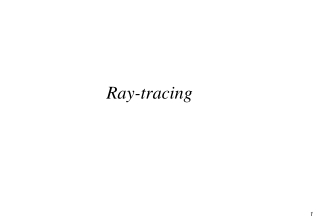DownloadDownload PresentationRay-tracing

# Ray-tracing

Télécharger la présentation## Ray-tracing

- - - - - - - - - - - - - - - - - - - - - - - - - - - E N D - - - - - - - - - - - - - - - - - - - - - - - - - - -
##### Presentation Transcript

1. Ray-tracing

2. Overview • Recursive Ray Tracing • Shadow Feelers • Snell’s Law for Refraction • When to stop!

3. Recap: Light Transport

4. ( ) m ( å ( ) ) M = + I k I k n l k h n + × × , r a i j d j s j = 1 j Recap: Local Illumination • Ambient, diffuse & specular components • The sum is over the specular and diffuse components for each light I a

5. Recap: Result of Ray Casting

6. Correcting for Non-Visible Lights N H E L surface

7. Where Sj is the result of intersecting the ray L with the scene objects • Note consider your intersection points along the ray L carefully • Hint – they might be beyond the light!

9. Recursive Ray-Tracing • We can simulate specular-specular transmission elegantly by recursing and casting secondary rays from the intersection points • We must obviously chose a termination depth to cope with multiple reflections

10. Introducing Reflection N H R • Where E L surface

11. Computing the reflection direction N E • Ideal reflection •  =  • R same plane as V and N R = aE + bN • E.N = N.R = a(N.E)+b • If a = -1, b = 2N.E R = -E + 2(N.E)N R  

12. Computing Reflectance • Where Ilocal is computed as before • Ray r' is formed from intersection point and the direction R and is cast into the scene as before

13. p’’ L2 R2 R1 p p’ L1 Recursive Ray Tracing

14. Pseudo Code Color RayTrace(Point p, Vector direction, int depth) { Point pd /* Intersection point */ Boolean intersection if (depth > MAX) return Black intersect(p,direction, &pd, &intersection) if (!intersection) return Background Ilocal = kaIa + Ip.v.(kd(n.l) + ks.(h.n)m) return Ilocal + kr*RayTrace(pd, R, depth+1) } Normally kr = ks

15. Result of Recursion

16. Perfect Specular Transmission N H E R Snell’s Law L  1 is index of refraction 2 T ß

17. Using Snell’s Law • Using this law it is possible to show that: • Note that if the root is negative then total internal reflection has occurred and you just reflect the vector as normal

18. T2 p’’ L2 R2 R1 p p’ T1 L1 Recursive Ray Tracing Including Transparent Objects

19. New Pseudo Code Color RayTrace(Point p, Vector D, int depth) { Point pd /* Intersection point */ Boolean intersection if (depth > MAX) return Black intersect(p,direction, &pd, &intersection) if (!intersection) return Background Ilocal = kaIa + Ip.v.(kd(n.l) + ks.(h.n)m) return Ilocal + kr*RayTrace(pd, R, depth+1) + kt*RayTrace(pd, T, depth+1) }

20. Direct Specular Transmission N • A transparent surface can be illuminated from behind and this should be calculated in Ilocal H' E L

21. Calculating H' • Use H‘ instead of H in specular term

22. Putting Everything Together

23. Discussion – What Can’t We Simulate?

24. Remark • Specular and transmission only • What should be added to consider diffuse reflection? • Why it’s expensive • Intersection of rays with polygons (90%) • How to reduce the cost? • Reduce the number of rays • Reduce the cost on each ray • First check with bounding box of the object • Methods to sort the scene and make it faster

25. Summary • Recursive ray tracing is a good simulation of specular reflections • We’ve seen how the ray-casting can be extended to include shadows, reflections and transparent surfaces • However this is a very slow process and still misses some types of effect!